# 978-0123869449 Chapter 19

Document Type
Homework Help
Book Title
Authors
Michael F. Modest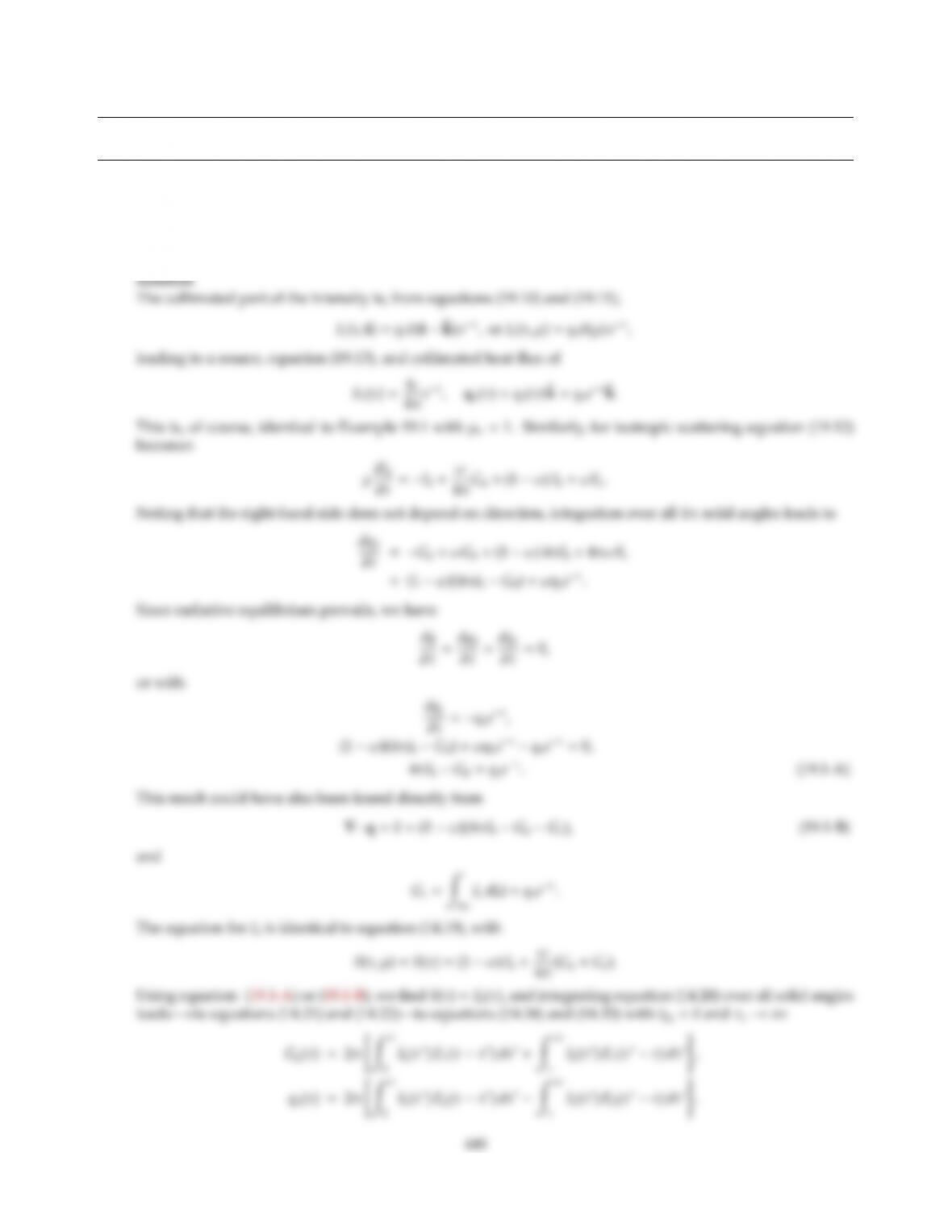CHAPTER 19
19.1 A semi-inﬁnite, gray, isotropically scattering medium, originally at zero temperature, is subjected to colli-
mated irradiation with a constant ﬂux qonormal to its nonreﬂecting surface. Set up the integral relationships
rium.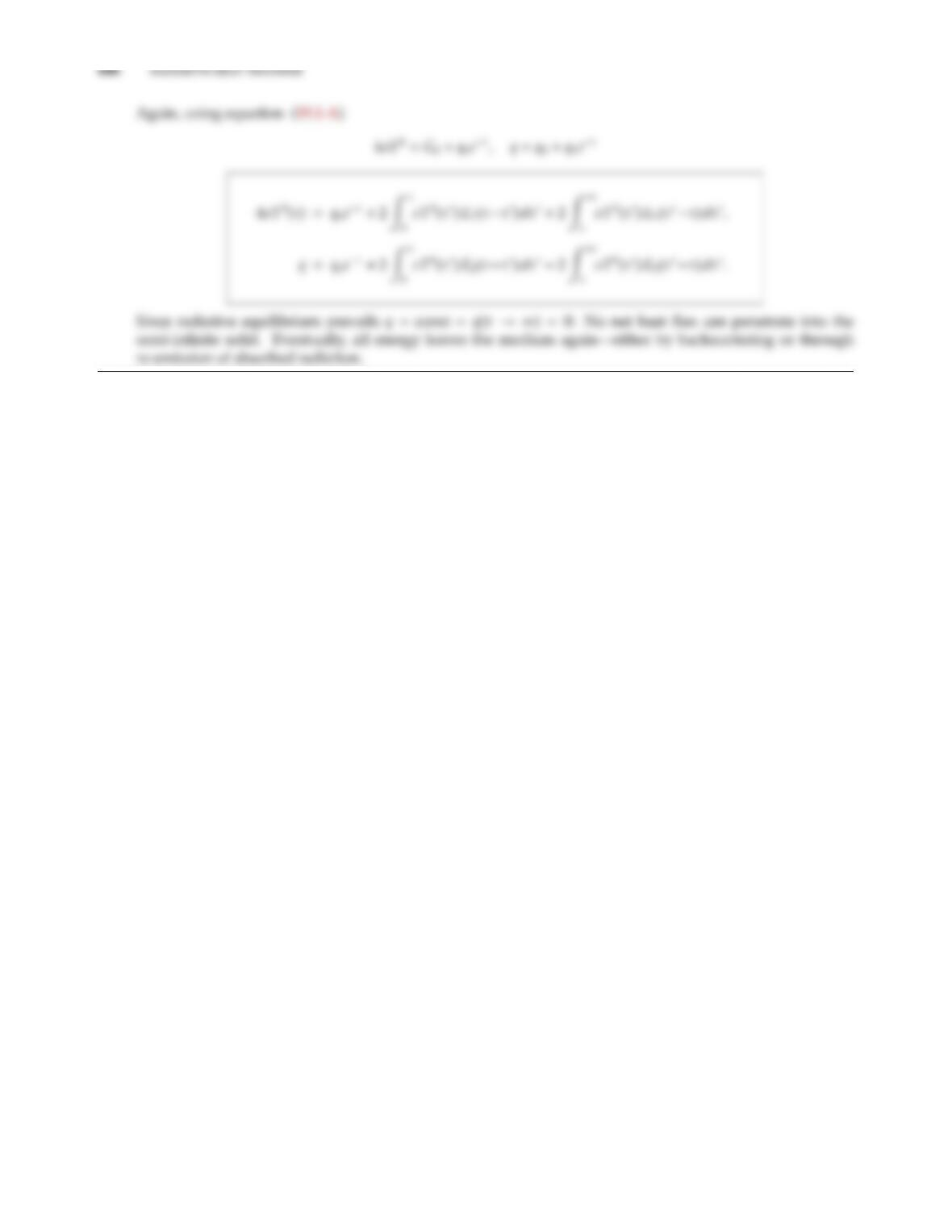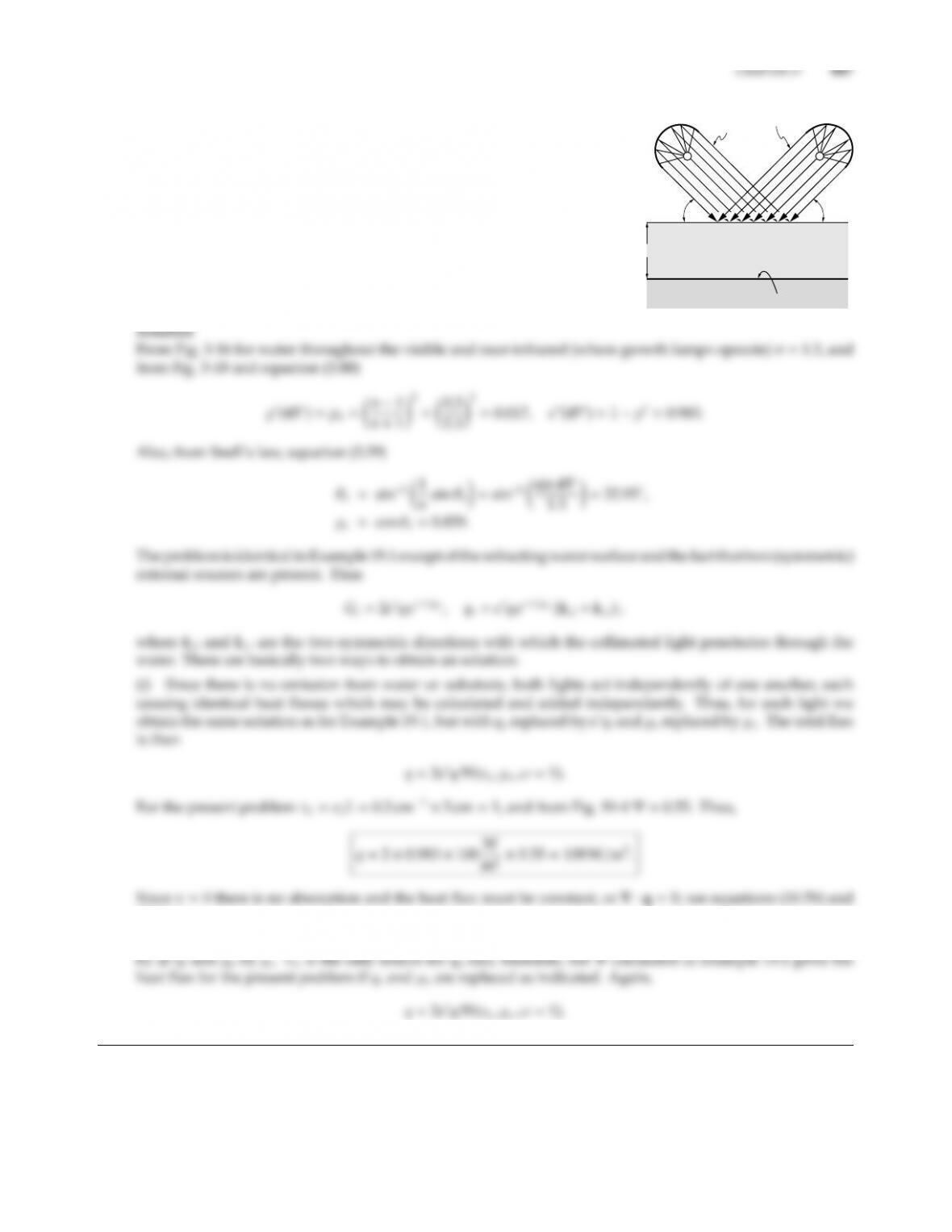19.2
L
ql
ql = 100W/m2
45° 45°
= 0,
s
σ
= 1
κ
= 0.2 cm–1
In a greenhouse a layer of water (thickness L=5 cm) is resting on
top of a black substrate. The water is loaded with growing organisms
that scatter light isotropically but do not absorb (σs=0.2 cm1). The
water layer is illuminated by two long growth-enhancing lights, ﬁtted
with reﬂector shields that make the light essentially parallel each light
delivering a heat ﬂux of ql=100 W/m2(per unit area normal to the
light rays). Using the exact method, calculate energy generated within
the water and the radiative heat ﬂux absorbed by the black surface in
the zone between the lights, where the heat transfer is essentially one-
dimensional. Emission from the water and substrate are negligible.
Hint: Use Figs. 3-16 and 19-4.
(10.61).
(ii) Alternatively, evaluating ˆ
qc·ˆ
kwe ﬁnd that Gcand ˆ
qc·ˆ
kare the same as in Example 19.1 if qsis replaced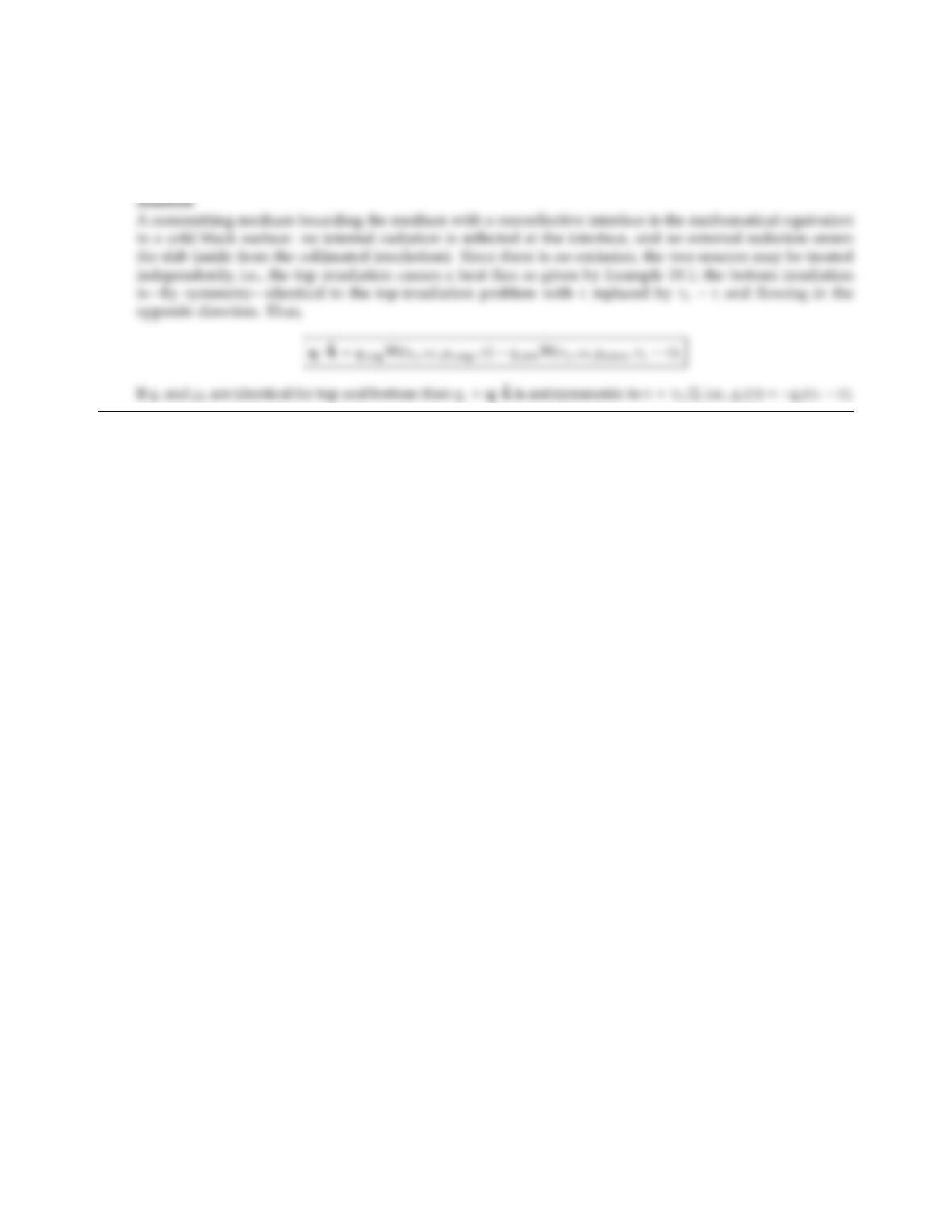19.3 Reconsider the medium described in Example 19.1. Rather than being bounded by a cold black surface at the
bottom, the layer is now exposed to the nonparticipating gas as well as to solar irradiation (using mirrors)
on both sides. Determine radiative heat ﬂux and its divergence within the layer in terms of the function
Φ(τL, ω, µo, τ) given in Example 19.1.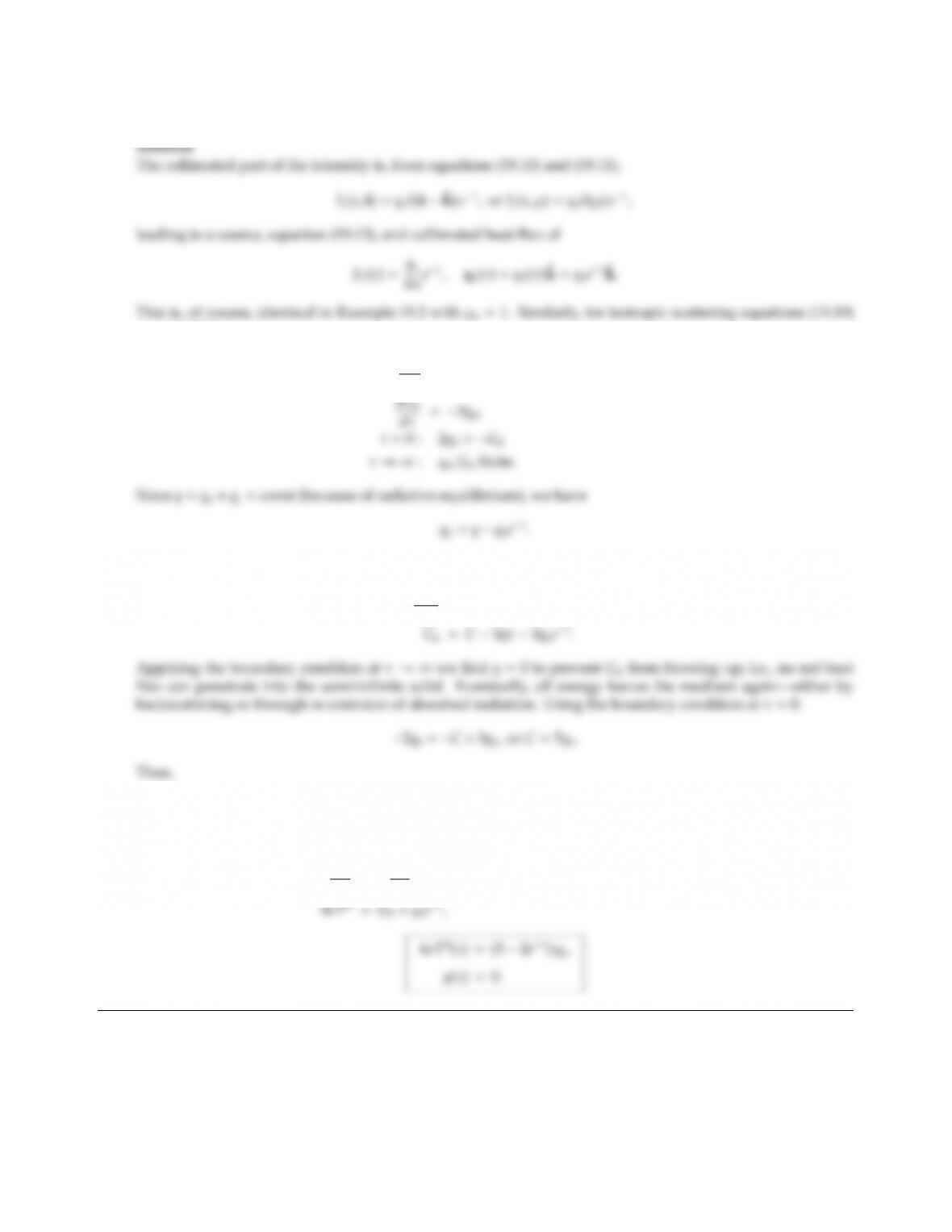CHAPTER 19 449
19.4 Solve Problem 19.1 using the P1-approximation.
and (19.20) become
dqd
dτ=(1 ω)(4σT4Gd)+ωGc,
Substituting,
dGd
dτ=3q+3qoeτ,
Gd=(5 3eτ)qo,
and
dqd
dτ=dqc
dτ=qoeτ=(1 ω)(4σT4Gd)+ωqoeτ,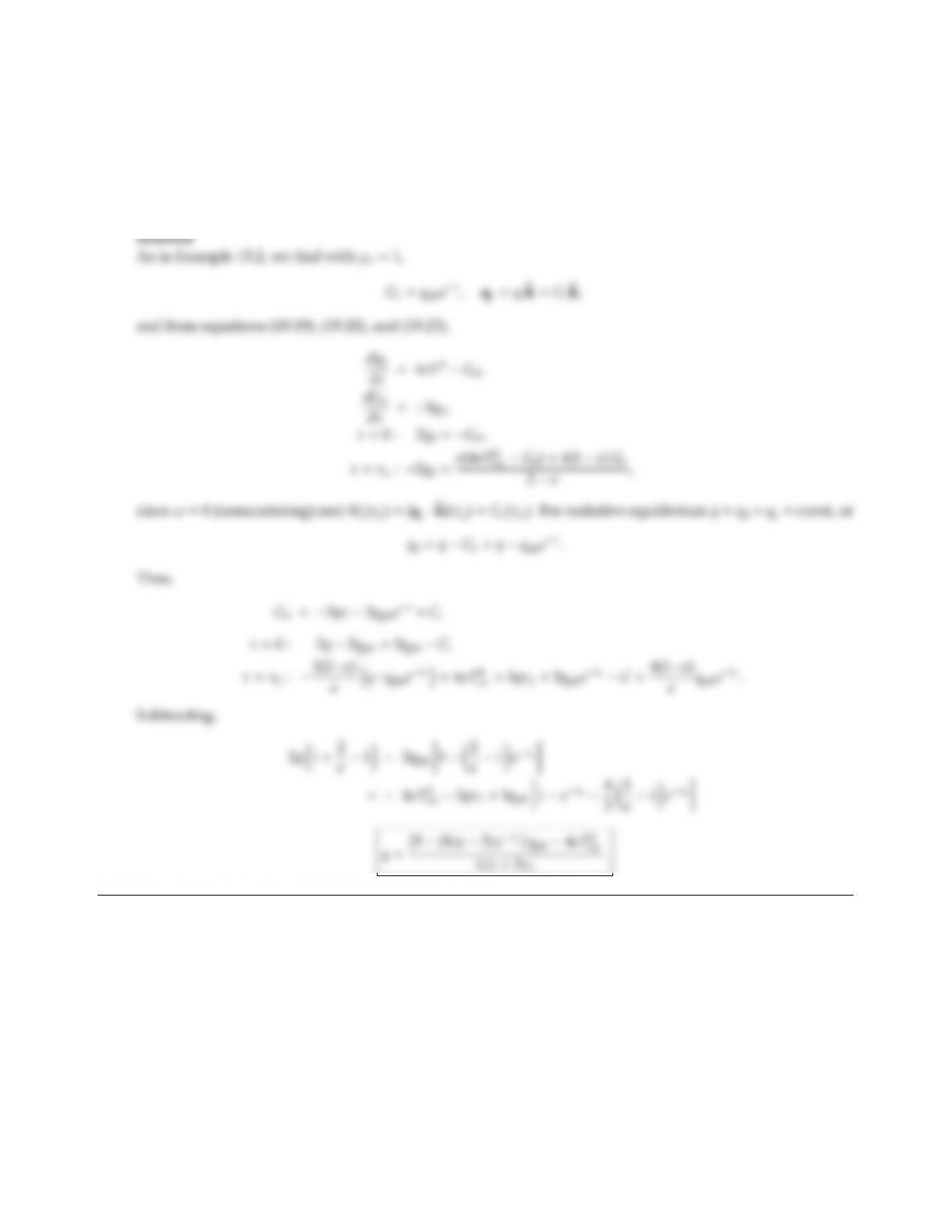19.5 The starship Enterprise is hitting a Klingon cruiser with its phaser gun. The armament of the cruiser is a
partially reﬂecting material that, after some irradiation, partly evaporates, forming a protective gas layer
above the surface. Assuming that the surface is at evaporation temperature Tev and has an emittance ǫ,
the gas has an absorption coecient κ1and a thickness L, determine the fraction of the heat ﬂux that hits
the Klingon ship. Under these conditions you may assume the eects of conduction and convection to be
negligible (but not reradiation from the gas). Use the P1-approximation.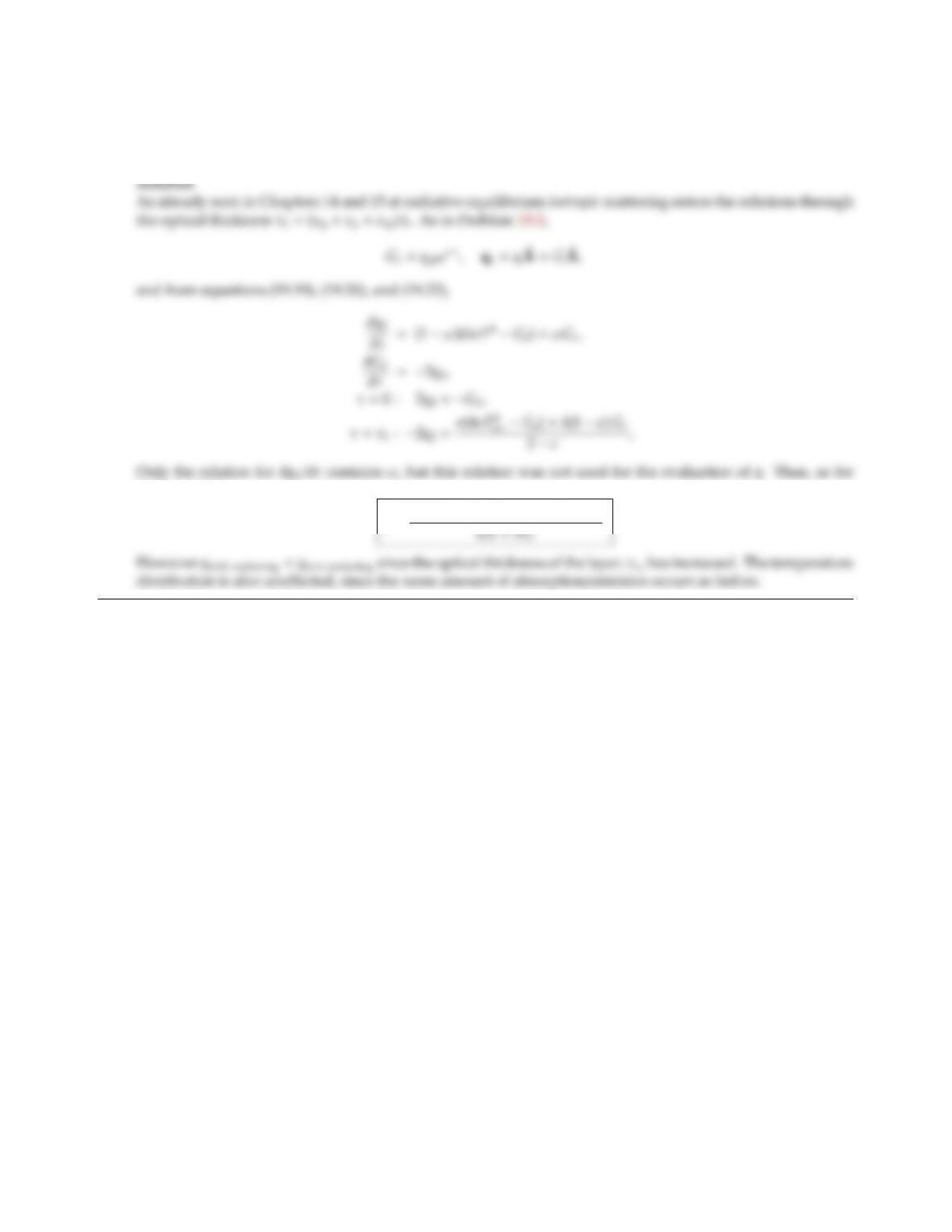CHAPTER 19 451
19.6 Reconsider Problem 19.5. After further irradiation, the surface material starts to disintegrate, spewing
particulate material into the gas layer. If we make the assumption that the debris has an absorption coecient
κpand (isotropic) scattering coecient σsp, how does this modify the surface irradiation?
Problem 19.5,
q=[5(83)eτL]qph 4σTev
.19.7 Consider a semi-inﬁnite gray medium with a nonreﬂecting surface. The medium is cold, absorbs (absorption
coecient κ) and scatters isotropically (scattering coecient σs). Collimated radiation obeying the relation
qc=qo(1 cos ατx)ˆ
k
shines normally onto the medium as shown. Determine the reﬂectivity of the medium (i.e., the fraction of the
irradiation leaving the interface in the opposite direction), using the P1-approximation.
Hint: To solve the two-dimensional governing equation, set Gd(τx, τz)=G1(τz)+G2(τz) cos ατx.
z, z
τ
x, x
τ
qc (x)
τ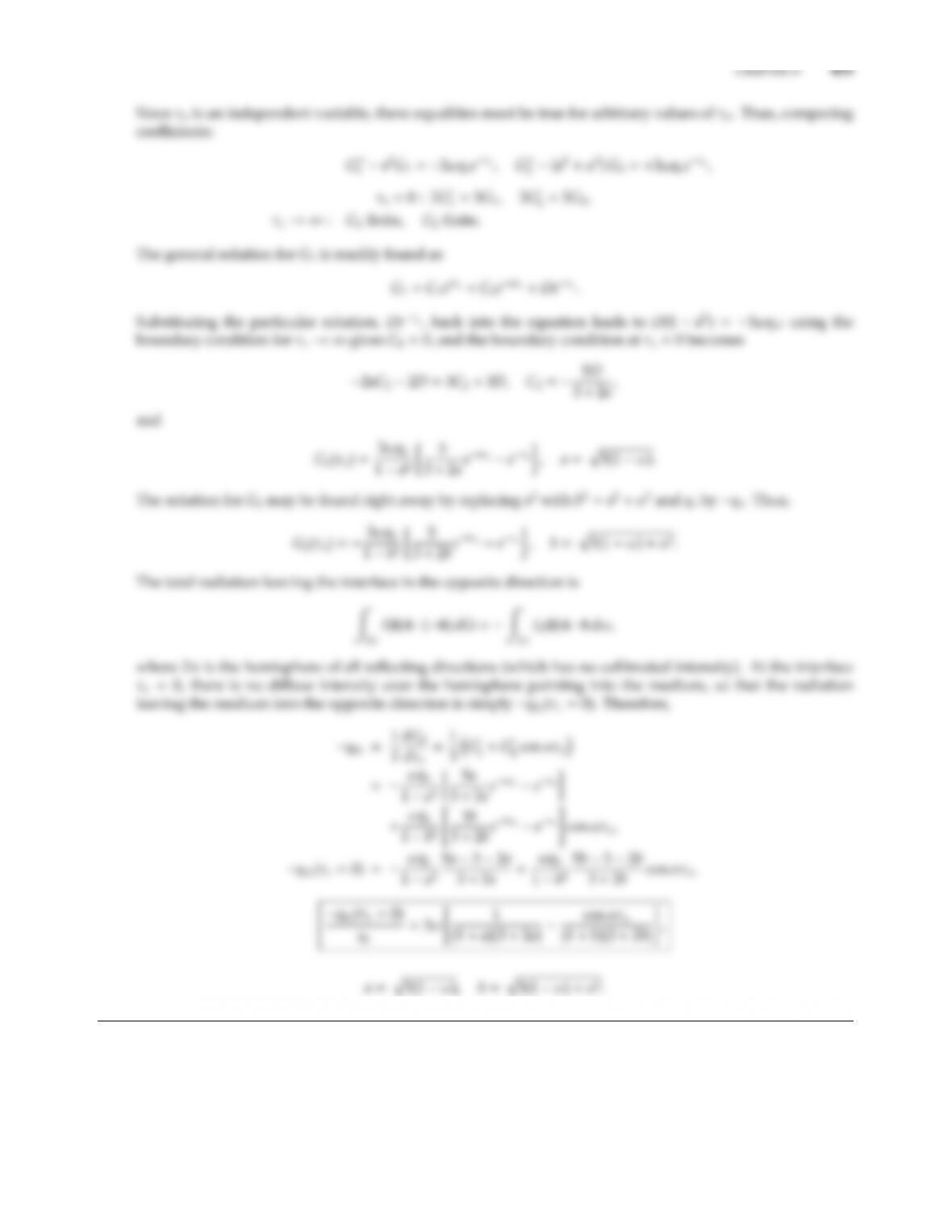## Trusted by Thousands ofStudents

Here are what students say about us.